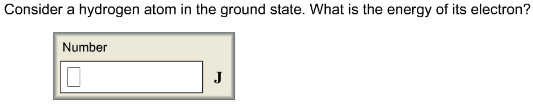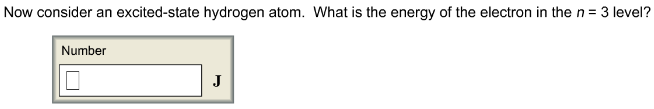# Consider a hydrogen atom in the ground state.What is the energy of its electron?

Consider a hydrogen atom in the ground state. What is the energy of its electron?the energy of electron in n^th level in hydrogen atom = -2.18*10^-18/n^2 J/atom

(A) in the ground state n=1

E = -2.1810^-18/1^2
E = -2.18
10^-18 J/atom

(B) in the ground state n=3

E = -2.1810^-18/3^2
E = -2.18
10^-18 J/atom
E = -2.42*10^-19 J/atom